# PL/SQL

### Nested Tables (and Data) in Oracle & ODM

Posted on

Oracle Data Mining uses Nested data types/tables to store some of its data. Oracle Data Mining creates a number of tables/objects that contain nested data when it is preparing data for input to the data mining algorithms and when outputting certain results from the algorithms.  In Oracle 11.2g there are two nested data types used and in Oracle 12.1c we get an additional two nested data types. These are setup when you install the Oracle Data Miner Repository. If you log into SQL*Plus or SQL Developer you can describe them like any other table or object.

DM_NESTED_NUMERICALS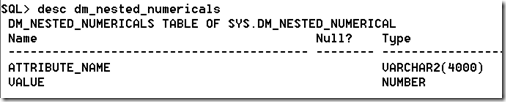DM_NESTED_CATEGORICALS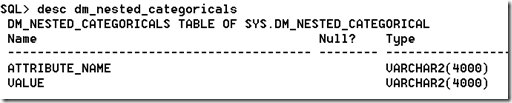The following two Nested data types are only available in 12.1c

DM_NESTED_BINARY_DOUBLES

DM_NESTED_BINARY_FLOATSThese Nested data types are used by Oracle Data Miner in preparing data for input to the data mining algorithms and for producing the some of the outputs from the algorithms.

Creating your own Nested Tables

To create your own Nested Data Types and Nested Tables you need to performs steps that are similar to what is illustrated in the following steps. These steps show you how to define a data type, how to create a nested table, how to insert data into the nested table and how to select the data from the nested table.

1. Set up the Object Type

Create a Type object that will defines the structure of the data. In these examples we want to capture the products and quantity purchased by a customer.

create type CUST_ORDER as object
(product_id     varchar2(6),
quantity_sold  number(6));
/

2. Create a Type as a Table

Now you need to create a Type as a table.

create type cust_orders_type as table of CUST_ORDER;
/

3. Create the table using the Nested Data

Now you can create the nested table.

create table customer_orders_nested (
cust_id       number(6) primary key,
order_date    date,
sales_person  varchar2(30),
c_order       CUST_ORDERS_TYPE)
NESTED TABLE c_order STORE AS c_order_table;

4. Insert a Record and Query

This insert statement shows you how to insert one record into the nested column.

insert into customer_orders_nested
values (1, sysdate, ‘BT’, CUST_ORDERS_TYPE(cust_order(‘P1’, 2)) );

When we select the data from the table we get

select * from customer_orders_nested;

CUST_ID ORDER_DAT SALES_PERSON
———- ——— ——————————
C_ORDER(PRODUCT_ID, QUANTITY_SOLD)
—————————————————–
1 19-SEP-13 BT
CUST_ORDERS_TYPE(CUST_ORDER(‘P1’, 2))

It can be a bit difficult to read the data in the nested column so we can convert the nested column into a table to display the results in a better way

select cust_id, order_date, sales_person, product_id, quantity_sold
from customer_orders_nested, table(c_order)

CUST_ID ORDER_DAT SALES_PERSON                   PRODUC QUANTITY_SOLD
———- ——— —————————— —— ————-
1 19-SEP-13 BT                             P1                 2

5. Insert many Nested Data items & Query

To insert many entries into the nested column you can do this

insert into customer_orders_nested
values (2, sysdate, ‘BT2’, CUST_ORDERS_TYPE(CUST_ORDER(‘P2’, 2), CUST_ORDER(‘P3’,3)));

When we do a Select * we get

CUST_ID ORDER_DAT SALES_PERSON
———- ——— ——————————
C_ORDER(PRODUCT_ID, QUANTITY_SOLD)
————————————————————-
1 19-SEP-13 BT
CUST_ORDERS_TYPE(CUST_ORDER2(‘P1’, 2))

2 19-SEP-13 BT2
CUST_ORDERS_TYPE(CUST_ORDER2(‘P2’, 2), CUST_ORDER2(‘P3’, 3))

Again it is not easy to ready the data in the nested column, so if we convert it to a table again we now get a row being displayed for each entry in the nested column.

select cust_id, order_date, sales_person, product_id, quantity_sold
from customer_orders_nested, table(c_order);

CUST_ID ORDER_DAT SALES_PERSON                   PRODUC QUANTITY_SOLD
———- ——— —————————— —— ————-
1 19-SEP-13 BT                             P1                 2
2 19-SEP-13 BT2                            P2                 2
2 19-SEP-13 BT2                            P3                 3

Advertisements

### 12c New Data Mining functions

Posted on

With the release of Oracle 12c we get new functions/procedures and some updated ones for Oracle Data Miner that is part of the Advanced Analytics option.

The following are the new functions/procedures and the functions/procedures that have been updated in 12c, with a link to the 12c Documentation that explains what they do.

• CLUSTER_DETAILS is a new function that predicts cluster membership for each row. It can use a pre-defined clustering model or perform dynamic clustering. The function returns an XML string that describes the predicted cluster or a specified cluster.

• CLUSTER_DISTANCE is a new function that predicts cluster membership for each row. It can use a pre-defined clustering model or perform dynamic clustering. The function returns the raw distance between each row and the centroid of either the predicted cluster or a specified.

• CLUSTER_ID has been enhanced so that it can either use a pre-defined clustering model or perform dynamic clustering.

• CLUSTER_PROBABILITY has been enhanced so that it can either use a pre-defined clustering model or perform dynamic clustering. The data type of the return value has been changed from `NUMBER` to `BINARY_DOUBLE`.

• CLUSTER_SET has been enhanced so that it can either use a pre-defined clustering model or perform dynamic clustering. The data type of the returned probability has been changed from `NUMBER` to `BINARY_DOUBLE`

• FEATURE_DETAILS is a new function that predicts feature matches for each row. It can use a pre-defined feature extraction model or perform dynamic feature extraction. The function returns an XML string that describes the predicted feature or a specified feature.

• FEATURE_ID has been enhanced so that it can either use a pre-defined feature extraction model or perform dynamic feature extraction.

• FEATURE_SET has been enhanced so that it can either use a pre-defined feature extraction model or perform dynamic feature extraction. The data type of the returned probability has been changed from `NUMBER` to `BINARY_DOUBLE`.

• FEATURE_VALUE has been enhanced so that it can either use a pre-defined feature extraction model or perform dynamic feature extraction. The data type of the return value has been changed from `NUMBER` to `BINARY_DOUBLE`.

• PREDICTION has been enhanced so that it can either use a pre-defined predictive model or perform dynamic prediction.

• PREDICTION_BOUNDS now returns the upper and lower bounds of the prediction as the `BINARY_DOUBLE` data type. It previously returned these values as the `NUMBER` data type.

• PREDICTION_COST has been enhanced so that it can either use a pre-defined predictive model or perform dynamic prediction. The data type of the returned cost has been changed from `NUMBER` to `BINARY_DOUBLE`.

• PREDICTION_DETAILS has been enhanced so that it can either use a pre-defined predictive model or perform dynamic prediction.

• PREDICTION_PROBABILITY has been enhanced so that it can either use a pre-defined predictive model or perform dynamic prediction. The data type of the returned probability has been changed from `NUMBER` to `BINARY_DOUBLE`.

• PREDICTION_SET has been enhanced so that it can either use a pre-defined predictive model or perform dynamic prediction. The data type of the returned probability has been changed from `NUMBER` to `BINARY_DOUBLE`.

### Part 3–Getting start with Statistics for Oracle Data Science projects

Posted on

This is the Part 3 blog post on getting started with Statistics for Oracle Data Science projects.

The table below is a collection of most of the statistical functions in Oracle 11.2. The links in the table bring you to the relevant section of the Oracle documentation where you will find a description of each function, the syntax and some examples of each.

 ABS LENGTH2 REGR_AVGX ACOS LENGTH4 REGR_ACGY Aggregrate functions LENGTHB REGR_COUNT Analytic functions LENGTHC REGR_INTERCEPT Arithmetic operators LN REGR_R2 ASIN LNNVL REGR_SLOPE ATAN LOG REGR_SXX ATAN2 LOWER REGR_SXY AVG LPAD REGR_SYY CAST LTRIM ROLLUP clause Comparison functions MAX ROUND CONCAT MEDIAN SAMPLE CORR MIN SIN CORR_K MOD SINH CORR_S MODEL clause SQRT COS NTH_VALUE STATS_BINOMIAL_TEST COSH Numeric Functions STATS_CROSSTAB COUNT PERCENT_RANK STATS_F_TEST COVAR_POP PERCENTILE_CONT STATS_KS_TEST COVAR_SAMP PERCENTILE_DISC STATS_MODE CUBE clause Pivot operations STATS_MW_TEST CUME_DIST POWER STATS_ONE_WAY_ANOVA CV PREDICTION STATS_T_TEST_INDEP Data functions PREDICTION_BOUNDS STATS_T_TEST_INDEPU DENSE_RANK PREDICTION_COST STATS_T_TEST_ONE EXP PREDICTION_PROBABILITY STATS_T_TEST_PAIRED FLOOR PREDICTION_SET STATS_WSR_TEST GREATEST PRESENTNNV STDDEV Grouping Sets PRESENTNTV STDEEV_POP INTERSECT Prior clause STDDEV_SAMP Interval arithmetic PRIOR SUM INTERVAL RANK TAN Julian dates RAWTOHEX TANH LAG REGEXP_COUNT t-test LAST REGEXP_INSTR VAR_POP LEAD REGEXP_LIKE VAR_SAMP LEAST REGEXP_REPLACE VARIANCE LENGTH REGEXP_SUBSTR WIDTH_BUCKET

The list about may not be complete (I’m sure it is not), but it will cover most of what you will need to use in your Oracle projects.

If you come across or know of other useful statistical functions in Oracle let me know the details and I will update the table above to include them.

### DBMS_PREDICTIVE_ANALYTICS & Explain

Posted on

There are 2 PL/SQL packages for performing data mining/predictive analytics in Oracle. The main PL/SQL package is DBMS_DATA_MINING. This package allows you to build data mining models and to apply them to new data. But there is another PL/SQL package.

The DBMS_PREDICTIVE_ANALYTICS package is very different to the DBMS_DATA_MINING package. The DBMS_PREDICTIVE_ANALYTICS package includes routines for predictive analytics, an automated form of data mining. With predictive analytics, you do not need to be aware of model building or scoring. All mining activities are handled internally by the predictive analytics procedure.

Predictive analytics routines prepare the data, build a model, score the model, and return the results of model scoring. Before exiting, they delete the model and supporting objects.

The package comes with the following functions: EXPLAIN, PREDICT and PROFILE. To get some of details about these functions we can run the following in SQL.This blog post will look at the EXPLAIN function.

EXPLAIN creates an attribute importance model. Attribute importance uses the Minimum Description Length algorithm to determine the relative importance of attributes in predicting a target value. EXPLAIN returns a list of attributes ranked in relative order of their impact on the prediction. This information is derived from the model details for the attribute importance model.

Attribute importance models are not scored against new data. They simply return information (model details) about the data you provide.

I’ve written two previous blog posts on Attribute Importance. One of these was on how to calculate Attribute Importance using the Oracle Data Miner tool. In the ODM tool it is now called Feature Selection and is part of the Filter Columns node and the Attribute Importance model is not persisted in the database.  The second blog post was how you can create the Attribute Importance using the DBMS_DATA_MINING package.

EXPLAIN ranks attributes in order of influence in explaining a target column.

The syntax of the function is

`DBMS_PREDICTIVE_ANALYTICS.EXPLAIN (     data_table_name     IN VARCHAR2,     explain_column_name IN VARCHAR2,     result_table_name   IN VARCHAR2,     data_schema_name    IN VARCHAR2 DEFAULT NULL);`
`where`

`data_table_name = `Name of input table or view

`explain_column_name = `Name of column to be explained

`result_table_name = `Name of table where results are saved. It creates a new table in your schema.

`data_schema_name = `Name of schema where the input table or view resides. Default: the current schema.

So when calling the function you do not have to include the last parameter.

Using the same example what I have given in the previous blog posts (see about for the links to these) the following command can be run to generate the Attribute Importance.

BEGIN

DBMS_PREDICTIVE_ANALYTICS.EXPLAIN(

data_table_name      => ‘mining_data_build_v’,

explain_column_name  => ‘affinity_card’,

result_table_name    => ‘PA_EXPLAIN’);

END;

One thing that stands out is that it is a bit slower to run than the DBMS_DATA_MINING method. On my laptop it took approx. twice to three time longer to run. But in total it was less than a minute.

To display the results,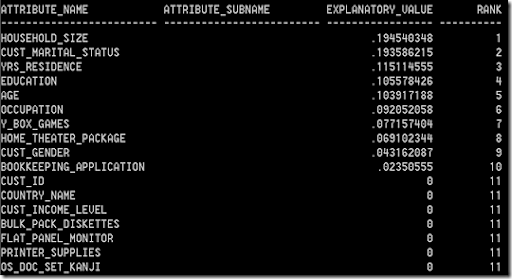The results are ranked in a 0 to 1 range. Any attribute that had a negative value are set to zero.

### Part 1–Getting started with Statistics for Oracle Data Science projects

Posted on

With all analytics or data science projects one of the first steps typically involves the extraction of data from various sources, merging the data and then performing various statistics.

The extraction and merging of the data is well covered by lots of other people blogging about how to use Oracle Data Integration (ODI), Oracle Warehouse Builder (OWB), among many others.

What I’m going to look at in this series of blog posts will be what statistical functions you might look at using in the Oracle and how to use them.

• This the first blog post in the series will look at the DBMS_STAT_FUNCS PL/SQL package, what it can be used for and I give some sample code on how to use it in your data science projects. I also give some sample code that I typically run to gather some additional stats.
• The second blog post will look at some of the other statistical functions that exist in SQL that you will/may use regularly in your data science projects.
• The third blog post will provide a summary of the other statistical functions that exist in the database.

These statistical functions can be grouped into 2 main types. The first is the descriptive statistics that are available by using the DBMS_STAT_FUNCS PL/SQL package and then there is the extensive list of other SQL stats functions.  It is worth mentioning at this point that all these stats packages and functions come as standard in the database (i.e. they are FREE, you do not have to pay for an add on option for the database to use them). So once you have you Oracle database installed you can start using them. There is no need to spend money buying another stats package to do stats. All you need to know is some SQL and what the stats functions are.

DBMS_STAT_FUNCS

One of the stats package that I use a lot is the SUMMARY function. This is part of the DBMS_STAT_FUNC PL/SQL package. This package calculates a number of common statistics for an attribute in a table. Yes that’s correct, it only gather statistics for just one attribute. So you will have to run it for all the numeric attributes in the table.

For does people who are familiar with the Oracle Data Miner tool, the explore data node produces a lot of these statistics that the SUMMARY function produces. See below for details of how to produce the Histograms.

The SUMMARY function has the following parameters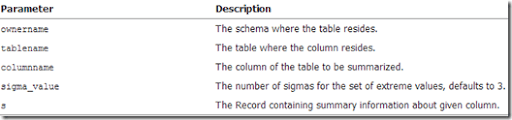Although you will probably be running this this function on the data in your schema you still have to give the schema name. The table name is the name of the table where the data exists, the column name is the name of the column that contains the actual data you want to analyse, and the ‘s’ is the record that will be returned by the SUMMARY function that contains all the summary information.

An example of the basic script to run the SUMMARY function is given below. It will use the data that is available in the sample schemas and the views that where setup for the Oracle Data Mining sample schemas. The table (or in this case the view) that we are going to use is the MINING_DATA_BUILD_V. What we are going to do is to replicate some of what the Explore Node does in the Oracle Data Miner tool.

set serveroutput on

declare
s         DBMS_STAT_FUNCS.SummaryType;
begin

DBMS_STAT_FUNCS.SUMMARY(‘DMUSER’, ‘MINING_DATA_BUILD_V’, ‘AGE’, 3, s);

dbms_output.put_line(‘SUMMARY STATISTICS’);
dbms_output.put_line(‘Count  : ‘||s.count);
dbms_output.put_line(‘Min    : ‘||s.min);
dbms_output.put_line(‘Max    : ‘||s.max);
dbms_output.put_line(‘Range  : ‘||s.range);
dbms_output.put_line(‘Mean   : ‘||round(s.mean));
dbms_output.put_line(‘Mode Count : ‘||s.cmode.count);
dbms_output.put_line(‘Mode        : ‘||s.cmode(1));
dbms_output.put_line(‘Variance    : ‘||round(s.variance));
dbms_output.put_line(‘Stddev      : ‘||round(s.stddev));
dbms_output.put_line(‘Quantile 5  : ‘||s.quantile_5);
dbms_output.put_line(‘Quantile 25 : ‘||s.quantile_25);
dbms_output.put_line(‘Median      : ‘||s.median);
dbms_output.put_line(‘Quantile 75 : ‘||s.quantile_75);
dbms_output.put_line(‘Quantile 95 : ‘||s.quantile_95);
dbms_output.put_line(‘Extreme Count : ‘||s.extreme_values.count);
dbms_output.put_line(‘Extremes      : ‘||s.extreme_values(1));
dbms_output.put_line(‘Top 5 : ‘||s.top_5_values(1)||’,’||
s.top_5_values(2)||’,’||
s.top_5_values(3)||’,’||
s.top_5_values(4)||’,’||
s.top_5_values(5));
dbms_output.put_line(‘Bottom 5 : ‘||s.bottom_5_values(5)||’,’||
s.bottom_5_values(4)||’,’||
s.bottom_5_values(3)||’,’||
s.bottom_5_values(2)||’,’||
s.bottom_5_values(1));
end;
/We can compare this to what is produced by the Explore Node in ODM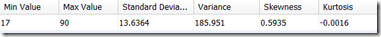We can see that the Explore Node gives us more statistics to help us with understanding the data.

What Statistics does the Explore Node produce

We can see the actual SQL code that the Explore Node runs to get the statistics that are displayed in the Explore Node View Data window. To do this you will need to right-click on the Explore Node and move the mouse down to the Deploy option. The submenu will open and select ‘SQL to Clipboard’ from the list. Open a text editor and past the code. You  will need to tidy up some of this code to point it at the actual data source you want. You will get the following

SELECT /*+ inline */  ATTR,
DATA_TYPE,
NULL_PERCENT,
DISTINCT_CNT,
DISTINCT_PERCENT,
MODE_VALUE,
AVG,
MIN,
MAX,
STD,
VAR,
SKEWNESS,
KURTOSIS,
HISTOGRAMS
FROM OUTPUT_1_23;

Where OUTPUT_1_23 is a working table that ODM has created to store intermediate results from some of its processing. In this case the Explore Node. You will need to change this to the ODM working table in your schema.

This query does not perform any of the statistics gathering. It just presents the results.

Creating our own Statistics gathering script – Part 1

The attribute names in the above SQL query tells us what statistics functions within Oracle that were used. We can replicate this statistics gathering task using the following script. There are four parts to this script. The first part gathers most of the common statistics for the attribute. The second and third parts calculate the Skewness and Kurtosis for the attribute. The final (fourth) part combines the first three parts and lists the outputs.

The one statistic function that we are not including at this point is the Histogram information. I will cover this in the next (second) blog post on statistics.

The following script has the data source table/view name included (MINING_DATA_BUILD_V) and the attribute we are going to use (AGE).  You will need to modify this script to run it for each attribute.

WITH
basic_statistics AS (select (sum(CASE WHEN age IS NULL THEN 1 ELSE 0 END)/COUNT(*))*100 null_percent,
count(*)    num_value,
count(distinct age)   distinct_count,
(count(distinct age)/count(*))*100     distinct_percent,
avg(age)      avg_value,
min(age)      min_value,
max(age)     max_value,
stddev(age)  std_value,
stats_mode(age)   mode_value,
variance(age)       var_value
from   mining_data_build_v),
skewness AS (select avg(SV) S_value
from (select power((age – avg(age) over ())/stddev(age) over (), 3) SV
from mining_data_build_v) ),
kurtosis AS (select avg(KV) K_value
from (select power((age – avg(age) over ())/stddev(age) over (), 4) KV
from mining_data_build_v) )
SELECT null_percent,
num_value,
distinct_percent,
avg_value,
min_value,
max_value,
std_value,
mode_value,
var_value,
S_value,
K_value
from basic_statistics,
skewness,
kurtosis;

Part 2 – Lets do it for all the attributes in a table

In the code above I’ve shown how you can gather the statistics for one particular attribute of one table.But in with an analytics project you will want to gather the statistics on all the attributes.

What we can do is to take the code above and put it into a procedure. This procedure accepts a table name as input, loops through the attributes for this table and calculates the various statistics. The statistics are saved in a table called DATA_STATS (see below).

drop table data_stats;

create table DATA_STATS (
table_name VARCHAR2(30) NOT NULL,
column_name VARCHAR2(30) NOT NULL,
data_type VARCHAR2(106) NOT NULL,
data_length NUMBER,
data_percision NUMBER,
data_scale NUMBER,
num_records NUMBER,
distinct_count NUMBER,
null_percent NUMBER,
distinct_percent NUMBER,
avg_value NUMBER,
min_value NUMBER,
max_value NUMBER,
std_value NUMBER,
mode_value VARCHAR2(1000),
var_value NUMBER,
s_value NUMBER,
k_value NUMBER,
PRIMARY KEY (table_name, column_name));

This is one of the first things that I do when I start on a new project. I create the DATA_STATS table and run my procedure GATHER_DATA_STATS for each table that we will be using. By doing this it allows me to have a permanent records of the stats for each attribute and saves me time in having to rerun various stats at different points of the project. I can also use these stats to produces some additional stats or to produce some graphs.

He is the code for the GATHER_DATA_STATS procedure.

CREATE OR REPLACE PROCEDURE gather_data_stats(p_table_name IN varchar2) AS

cursor c_attributes (c_table_name varchar2)
is SELECT table_name,
column_name,
data_type,
data_length,
data_precision,
data_scale
FROM user_tab_columns
WHERE table_name = upper(c_table_name);

v_sql     NUMBER;
v_rows    NUMBER;
BEGIN
dbms_output.put_line(‘Starting to gather statistics for ‘||upper(p_table_name)||’ at ‘||to_char(sysdate,’DD-MON-YY HH24:MI:SS’));

FOR r_att in c_attributes(p_table_name) LOOP
—
— remove any previously generated stats
—
v_sql := DBMS_SQL.OPEN_CURSOR;
DBMS_SQL.PARSE(v_sql, ‘delete from DATA_STATS where table_name = ”’||r_att.table_name||”’ and column_name = ”’||r_att.column_name||””, DBMS_SQL.NATIVE);
v_rows := DBMS_SQL.EXECUTE(v_sql);
–dbms_output.put_line(‘delete from DATA_STATS where table_name = ”’||r_att.table_name||”’ and column_name = ”’||r_att.column_name||””);

IF r_att.data_type = ‘NUMBER’ THEN
dbms_output.put_line(r_att.table_name||’ : ‘||r_att.column_name||’ : ‘||r_att.data_type);

—
— setup the insert statement and execute
—
v_sql := DBMS_SQL.OPEN_CURSOR;
DBMS_SQL.PARSE(v_sql, ‘insert into data_stats select ”’||r_att.table_name||”’, ”’||r_att.column_name||”’, ”’||r_att.data_type||”’, ‘||r_att.data_length||’, ‘||nvl(r_att.data_precision,0)||’, ‘||nvl(r_att.data_scale,0)||’, count(*) num_value, (sum(CASE WHEN ‘||r_att.column_name||’ IS NULL THEN 1 ELSE 0 END)/COUNT(*))*100 null_percent, count(distinct ‘||r_att.column_name||’) distinct_count, (count(distinct ‘||r_att.column_name||’)/count(*))*100 distinct_percent, avg(‘||r_att.column_name||’) avg_value, min(‘||r_att.column_name||’) min_value, max(‘||r_att.column_name||’) max_value, stddev(‘||r_att.column_name||’) std_value, stats_mode(‘||r_att.column_name||’) mode_value, variance(‘||r_att.column_name||’) var_value, null, null from ‘|| r_att.table_name, DBMS_SQL.NATIVE);
v_rows := DBMS_SQL.EXECUTE(v_sql);

ELSIF r_att.data_type IN (‘CHAR’, ‘VARCHAR’, ‘VARCHAR2’) THEN
dbms_output.put_line(r_att.table_name||’ : ‘||r_att.column_name||’ : ‘||r_att.data_type);

—
— We need to gather a smaller number of stats for the character attributes
—
v_sql := DBMS_SQL.OPEN_CURSOR;
begin

DBMS_SQL.PARSE(v_sql, ‘insert into data_stats select ”’||r_att.table_name||”’, ”’||r_att.column_name||”’, ”’||r_att.data_type||”’, ‘||r_att.data_length||’, ‘||nvl(r_att.data_precision,0)||’, ‘||nvl(r_att.data_scale,0)||’, count(*) num_value, (sum(CASE WHEN ‘||r_att.column_name||’ IS NULL THEN 1 ELSE 0 END)/COUNT(*))*100 null_percent, count(distinct ‘||r_att.column_name||’) distinct_count, (count(distinct ‘||r_att.column_name||’)/count(*))*100 distinct_percent, null, null, null, null, stats_mode(‘||r_att.column_name||’) mode_value, null, null, null from ‘|| r_att.table_name, DBMS_SQL.NATIVE);
v_rows := DBMS_SQL.EXECUTE(v_sql);

— dbms_output.put_line(‘insert into data_stats select ”’||r_att.table_name||”’, ”’||r_att.column_name||”’, ”’||r_att.data_type||”’, ‘||r_att.data_length||’, ‘||nvl(r_att.data_precision,0)||’, ‘||nvl(r_att.data_scale,0)||’, count(*) num_value, (sum(CASE WHEN ‘||r_att.column_name||’ IS NULL THEN 1 ELSE 0 END)/COUNT(*))*100 null_percent, count(distinct ‘||r_att.column_name||’) distinct_count, (count(distinct ‘||r_att.column_name||’)/count(*))*100 distinct_percent, null, null, null, null, stats_mode(‘||r_att.column_name||’) mode_value, null, null, null from ‘|| r_att.table_name);
exception
when others then
dbms_output.put_line(v_rows);
end;

ELSE
dbms_output.put_line(‘Unable to gather statistics for ‘||r_att.column_name||’ with data type of ‘||r_att.data_type||’.’);
END IF;
END LOOP;

dbms_output.put_line(‘Finished gathering statistics for ‘||upper(p_table_name)||’ at ‘||to_char(sysdate,’DD-MON-YY HH24:MI:SS’));

commit;
END;

Then to run it for a table:

exec gather_data_stats(‘mining_data_build_v’);

We can view the contents of the DATA_STATS table by executing the following in SQL*Plus or SQL Developer

select * from DATA_STATS;### OUG Norway April 2013 – New Year’s News

Posted on

I received an email at 23:24 on the 1st January from the OUG in Norway telling me that I’ve had two presentations accepted for the Annual OUG Norway seminar event. This will be on during the 17th-19th April.

The first day of this event (17th April) will be held in a hotel in Oslo. Then on the morning of 18th April we board the Color Magic cruise for the next two days of the conference. The ferry/cruise will go from Oslo to Kiel in Germany and then back again to Oslo, returning around 10am on Saturday 20th April.

I will be giving two presentations on the Oracle Advanced Analytics Option. The first presentation, ‘Using Predictive Analytics in Oracle’, will give an overview of the Oracle Advanced Analytics Option and will then focus on the Oracle Data Miner work-flow tool. This will presentation will include a live demo of using Oracle Data Miner to create some data mining models.

The second presentation, ‘How to Deploy and Use your Oracle Data Miner Models in Production’, builds on the examples given in the first presentation and will show how you can migrate, user and update your Oracle Data Miner models using the features available in SQL and PL/SQL. Again a demo will be given.

### Update on : Adding numbers between

Posted on

Over the past few days I’ve had a number of emails and comments based on my previous post.  My previous post was called ‘Adding numbers between two values’. I included some PL/SQL code that can be used to add up the numbers between two values. I mentioned that this was a question that my pre-teen son (a few year pre-teen) had asked me.

There are two main solutions to the same problem. One involves just using a SELECT and the other involves using recursion. I will come back the these alternative solutions below.

But let me start off with a bit more detail and background to why I approached the problem the way that I did. The main reason is that my son is a pre-teen. Over the past couple of years he as expressed an interest in what his daddy does. We even have matching ORACLENERD t-shirtsWhen I was working through the problem with my son I wanted to show him how to take a problem and by breaking it down into its different parts we can work out an overall solution. We can then take each of these parts and translate them into code. In this case some PL/SQL, yes it is a bit nerdy and we do have the t-shirt. The code that I gave illustrates many different parts of the language and hopefully he will use some of these features as we continue on our learning experience.

It is good sometimes to break a problem down into smaller parts. That way we can understand it better, what works and what does not work, if something does not work then we will know what bit and also leads to easier maintenance. At a later point as you develop an in-depth knowledge of certain features of a language you can then rewrite what you have to be more efficient.

All part of the learning experience.

Ok lets take a look at the other ways to answer this problem. The first approach is to just use a single SELECT statement.

SELECT sum(rownum + &&Start_Number – 1)
FROM    dual
CONNECT by level <= &End_Number – &&Start_Number + 1;

An even simpler way is

SELECT sum(level)
FROM    dual
CONNECT BY level between &Start_Number and &End_Number;

These queries create a hierarchical query that produce all the numbers between the Start_Number parameter and the End_Number parameter. The SUM is needed to all all the numbers/rows produced.  This is nice and simple (but not that easy for by son at this point).

Thank you to everyone who contacted me about this. I really appreciated your feedback and please keep your comments coming for all my posts.